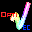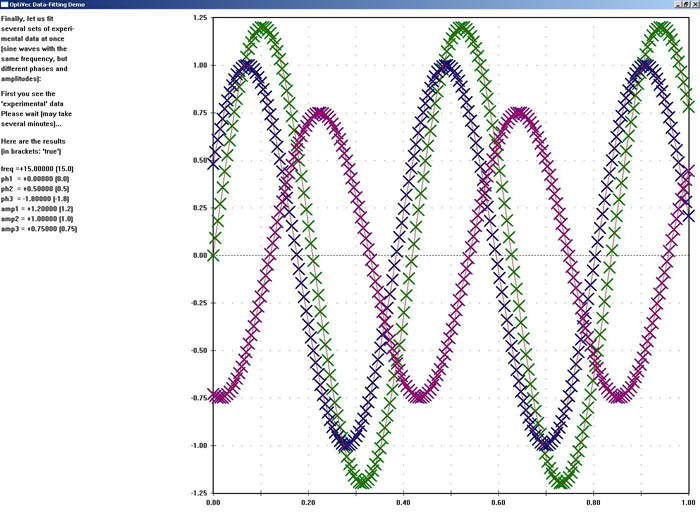# OptiVec for Delphi

8.0.1
OptiVec: Fast vector and matrix library for DelphiVector and matrix library for Delphi
OptiVec contains more than 3500 hand-optimized, Assembler-written functions for all floating-point and integer data types from the following fields: 1. Vectorized form of arithmetic operators and math functions. 2. Matrix operations, e.g.: multiplication, inversion, LU decomposition, singular value decomposition, Cholesky, eigenvalues. 3. Fast Fourier Transform techniques for efficient convolutions, correlation analyses, spectral filtering, etc., both one- and two-dimensional. 4. Curve fitting for a wide range of model functions from simple linear regression to non-linear models with multiple data sets. 5. Statistics. 6. Comparisons (e.g., as building blocks for time series analysis). 7. Analysis (derivatives, integrals, extrema, interpolation). 8. Graphical representation of data in Cartesian coordinates. 9. Complex number math, both in cartesian and polar format. The vectorized implementation in Assembler makes OptiVec functions, on the average, 2-3 times faster than compiled source code of the same functionality. In many instances, the numerical accuracy is improved as well. This version contains the units for all Delphi versions (Borland Delphi, Embarcadero RAD Studio) from Delphi 7 to XE6 (except Delphi 8), both for 32-bit and for 64-bit (Delphi XE2 or higher).
Technical details
Title:
OptiVec for Delphi 8.0.1 from Windows
Requirements:
Any version of Delphi or RAD Studio from Delphi 7 to the XE series and 10.x Seattle / Berlin / Tokyo
OS Support:
Win2000, WinXP, Win7 x32, Win7 x64, Windows 8, Windows 10, WinServer, WinOther, WinVista, WinVista x64
Language:
German, English